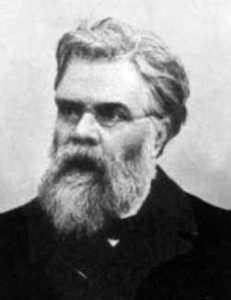# Koch’s Snowflake in Python

```# Draw a Koch snowflake
from turtle import *

def koch(a, order):
if order > 0:
for t in [60, -120, 60, 0]:
forward(a/3)
left(t)
else:
forward(a)

# Test
koch(100, 0)
pensize(3)
koch(100, 1)
```

Now, we make a small change to the function koch:

```    for t in [60, -120, 60, 0]:
koch(a/3, order-1)
left(t)
```

Completed code:

```# Choose colours and size
color("sky blue", "white")
bgcolor("black")
size = 400
order = 0

# Ensure snowflake is centred
penup()
backward(size/1.732)
left(30)
pendown()

# Make it fast
tracer(100)
hideturtle()

begin_fill()

# Three Koch curves
for i in range(3):
koch(size, order)
right(120)

end_fill()

# Make the last parts appear
update()
```

Source.

```
x = int(input("What is the number to square root?"))
for i in range(2,100):
if x%(i**2) == 0:
x = int(x/i**2)

```

# A Biography of Émile LemoineIn the link below, a brief biography of mathematician Emile Lemoine, responsible for the Lemoine point of a triangle and Lemoine’s Conjecture, sometimes attributed to the later work of Hyman Levy.  Lemoine’s Conjecture is a stronger form of the Goldbach Conjecture.

Biography: Emile-Michel-Hyacinthe Lemoine
Author(s): David Eugene Smith
Source: The American Mathematical Monthly, Vol. 3, No. 2 (Feb., 1896), pp. 29-33